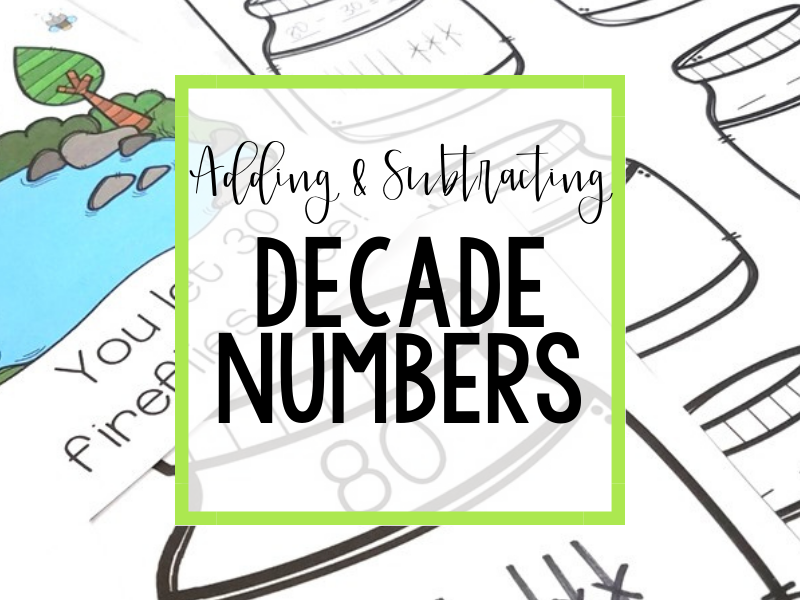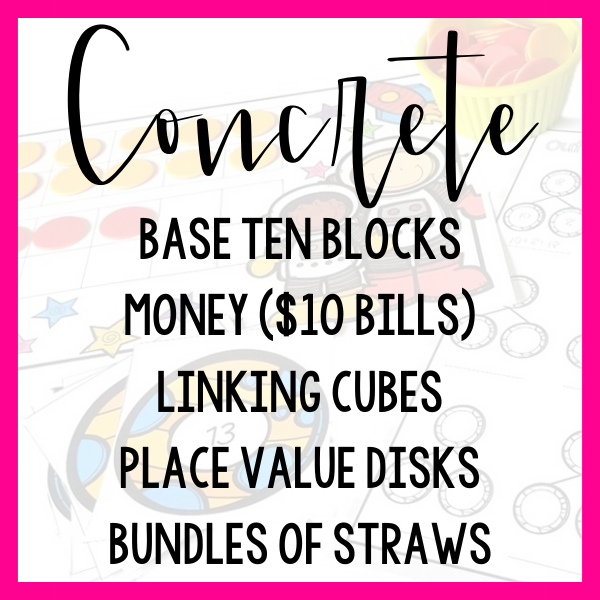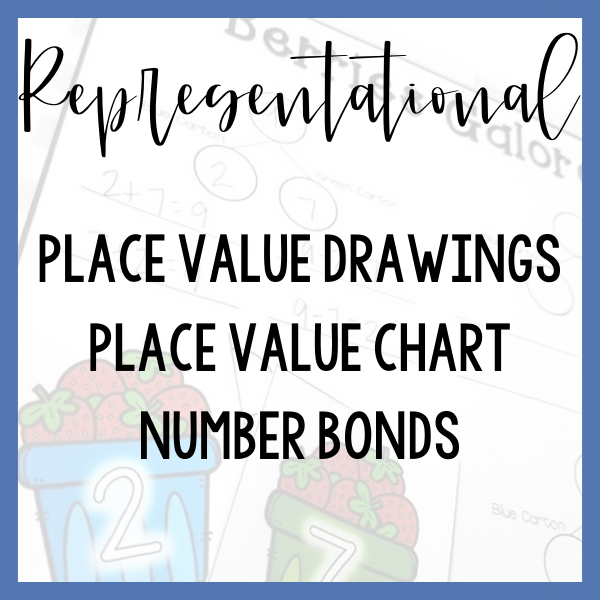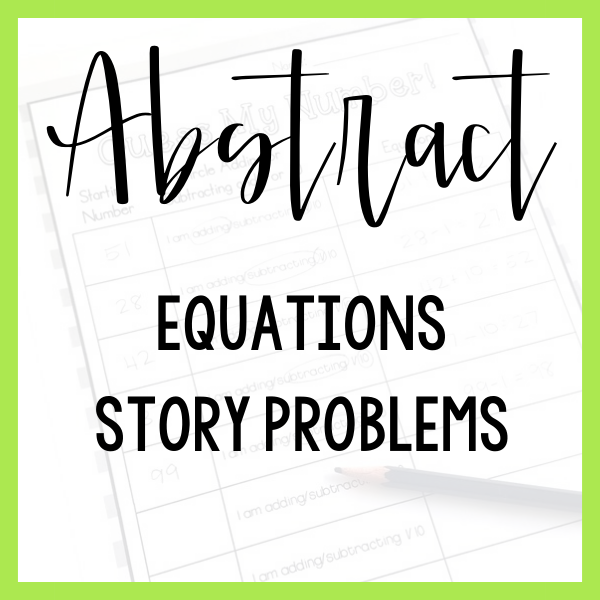First graders begin to solve addition and subtraction problems with sums to 100. It is SO important to note, these standards are NBT (place value) standards, not OA (operations) standards! Noting this will help you to stay focused as you are instructing and remediating. This instruction is all about place value!The 5 examples listed are certainly not an exhaustive list of tools and lessons that could be used to teach this skill.

They are rather a sample progression from hands-on to abstract thinking!

Which step represents your students’ current level of understanding?

Don’t start these lessons taking for granted the fact that your students know that ten ones makes a ten. This is still an emerging understanding for students learning to work with decade numbers!

Give your students a pile of cubes (unlinked!) in two colors. For example, 20 red cubes and 30 blue cubes.

Ask your students if they can find the total number of cubes.

Is there a way they might organize their cubes to make them easier to count?

Draw your students attention to the number of ten sticks they were able to make with their cubes.

Record equations that describe how they found the total in multiple ways. 20 + 30 = 50 and also 2 tens + 3 tens = 5 tens.

Repeat the activity many times so your students have the chance to draw connections between ten ones and “a ten”.

Much in the same way you explored addition, you can support your students to explore subtraction!

Begin by using the same tool (linking cubes) that you used when adding decade numbers but, as your students begin to gain confidence around ten ones making a ten, you have the opportunity to move to a pregrouped model such as base ten blocks or even \$10 bills!

Continue to record equations both in standard form (60 – 40 = 20) and in place value form (6 tens – 4 tens = 2 tens).

Using place value form helps to connect to the basic addition and subtraction facts that your students know.

To help your students solidify their understanding and also to prevent overgeneralizations, include time for students to explore addition and subtraction scenarios side by side.

Story problems can be an excellent tool to support your students in truly thinking about whether numbers need to be put together or taken apart.

Provide your students with a variety of hands-on tools to choose from as they work. Linking cubes, base ten blocks, place value disks, \$10 bills or even bundles of 10 straws are all useful tools.

To take a step towards abstract understanding and away from hands-on tools, support your students in using place value drawings to add or subtract decade numbers.

Ask your students to model a scenario using base ten blocks- they look a lot like a place value drawing! As your students model with blocks, demonstrate how you can show exactly the same action using a place value drawing.

Moving to a less supportive model (such as hands-on to representational drawings) is all about linking what your students know with the new model!

We know one of the strongest methods for strengthening our students ability to subtract is by supporting them in relating addition and subtraction!

Provide your students with a number bond filled with numbers such as 70, 40 and 30. Ask your students how they might create addition and subtraction equations using these numbers.

You can use the “walk backwards” method to give your students only as much support as they need.

Start by asking your students to write an equation that could be created with these three numbers. Walk backwards to representational models- could they draw a place value drawing to match their equation?

Walk backwards one more time- could your students build a model using math tools to match their place value drawing?

Along the way, allow your students to adjust their equation if they find that their place value drawing or hands-on model changes their thinking.

# Tools To USe